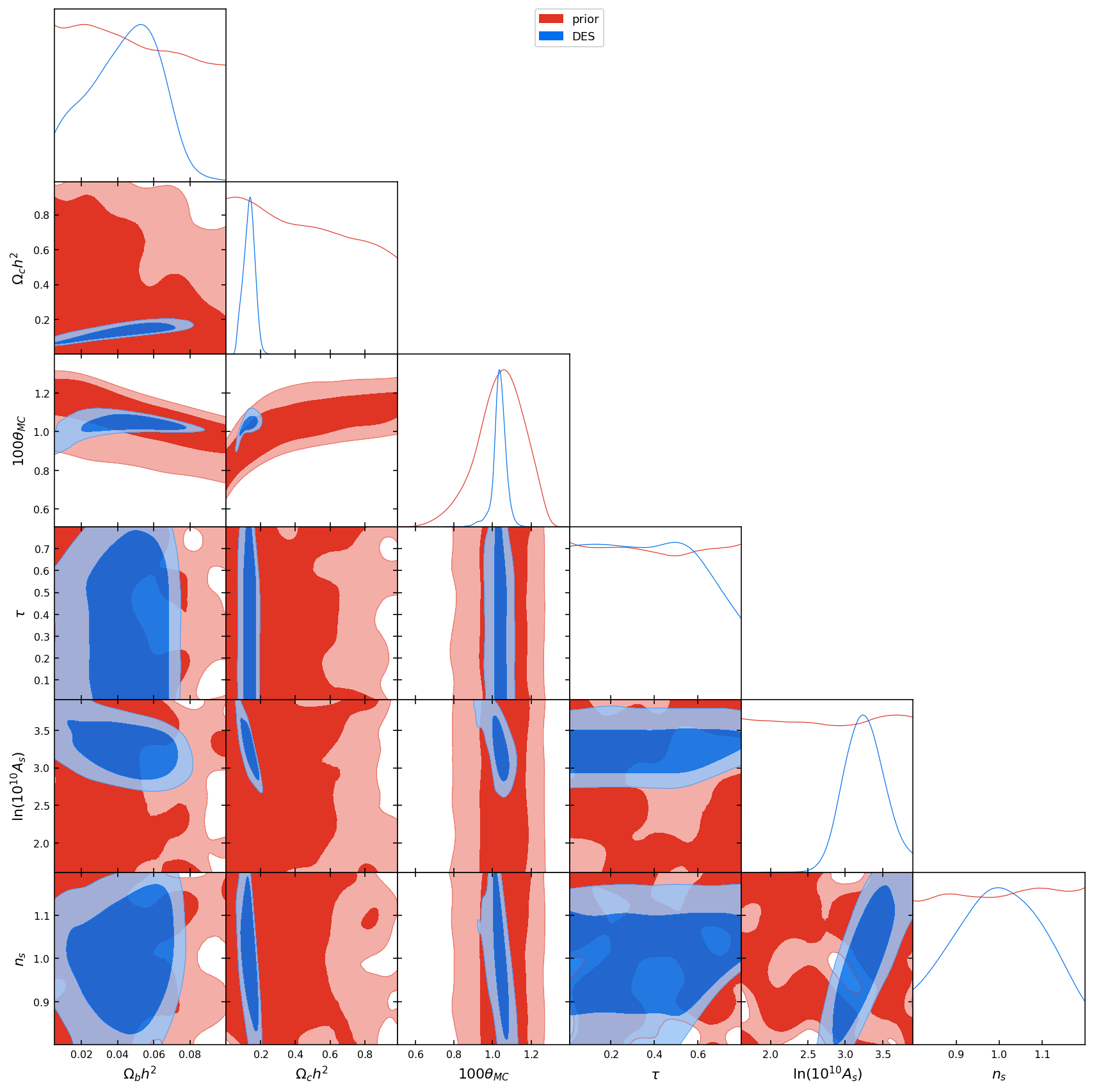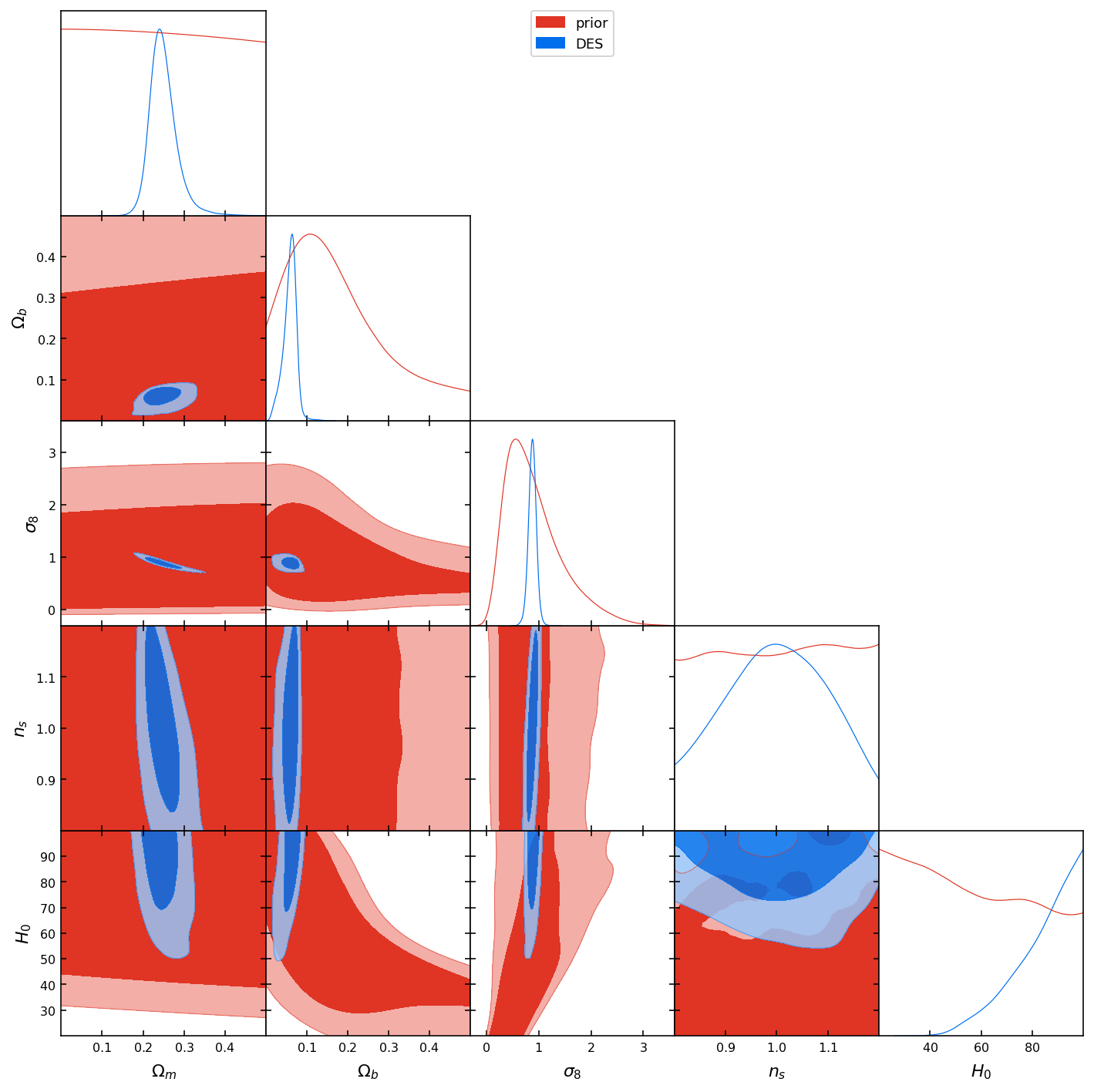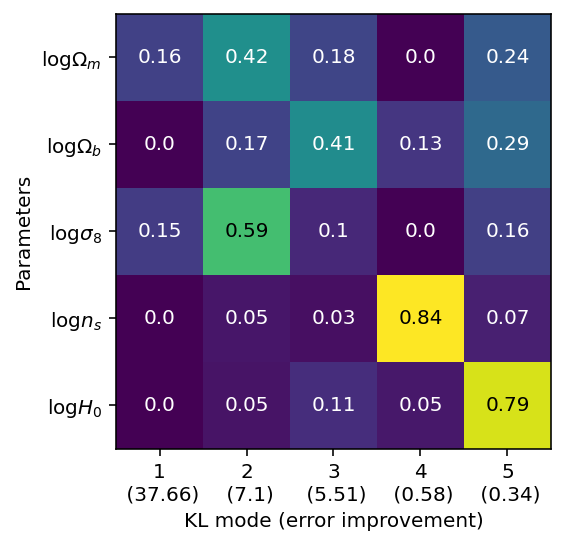# What is an experiment measuring?¶

Marco Raveri (mraveri@sas.upenn.edu)

In this notebook we show a worked example of how to go from the posterior of a given experiment to understanding the physics that controls its best constrained properties.

We work with the results of the Dark Energy Survey (DES) first year of data (https://arxiv.org/abs/1708.01530). Specifically we consider the full weak lensing and galaxy clustering (3x2) analysis.

In :
# Show plots inline, and load main getdist plot module and samples class
%matplotlib inline
%config InlineBackend.figure_format = 'retina'
# import libraries:
import sys, os
sys.path.insert(0,os.path.realpath(os.path.join(os.getcwd(),'../..')))
from getdist import plots, MCSamples
from getdist.gaussian_mixtures import GaussianND
import getdist
import scipy
import matplotlib.pyplot as plt
import IPython
from IPython.display import Markdown
import numpy as np
import seaborn as sns
# import the tensiometer tools that we need:
from tensiometer import utilities
from tensiometer import gaussian_tension


We now import the chains with the parameter posteriors.

Notice that we have removed many parameters from the chains since they were irrelevant to the example. The chains are already fully polished. Burn in has been removed and the samples have been thinned (to save space on github).

We have run a prior only chain to ensure that the modeling of the prior is as faithful as possible. In particular, in standard cosmological analyses, we have priors on derived parameters that would give non-trivial shapes to the parameters that are being sampled (see for example Appendix F in https://arxiv.org/abs/1806.04649)

In :
# load the chains (remove no burn in since the example chains have already been cleaned):
chains_dir = './../../test_chains/'
# the DES Y1 3x2 chain:
settings = {'ignore_rows':0, 'smooth_scale_1D':0.3, 'smooth_scale_2D':0.3}
# the prior chain:
# we add a couple of derived parameters that have been removed from the chains (to save a little space on github):
for ch in [chain, prior_chain]:
p = ch.getParams()


Now we look at the parameters that were used to run the chain and we plot them against the prior:

In :
param_names = chain.getParamNames().getRunningNames()
g = plots.get_subplot_plotter()
g.triangle_plot([prior_chain, chain], params=param_names, filled=True)

/Users/marco/Software/anaconda3/lib/python3.7/site-packages/getdist/plots.py:1084: UserWarning: No contour levels were found within the data range.
alpha=alpha * self.settings.alpha_factor_contour_lines, **clean_args(kwargs))This plot is not particularly illuminating. We might guess that 4 parameteres are constrained but it is hard to be sure about the number because of degeneracies.

## How many parameters are constrained?¶

To quantify how many parameters DES is measuring over the prior we compute the number of constrained parameters, as discussed in (https://arxiv.org/pdf/1806.04649.pdf).

The posterior covariance, $C_p$, combines the prior, $C_\Pi$, and data, $C$, covariances as: \begin{align} C_p^{-1} = C_\Pi^{-1} + C^{-1} \end{align} A parameter is measured when the posterior is closest to the purely data covariance $C^{-1}C_p \sim I$ (i.e. the prior is irrelevant).

With a little algebra the number of parameters that are constrained by the data over the prior is then: \begin{align} N_{\rm eff} \equiv N -{\rm Tr}(C_\Pi^{-1} C_p) \end{align} where $N$ is the nominal number of parameters.

This tells us how many parameters the data set is measuring. Notice that one important property is that this quantity is reparametrization invariant so it does not really depend on the specific parameters that we are using.

In :
gaussian_tension.get_Neff(chain, param_names=param_names, prior_chain=prior_chain)

Out:
3.314772623683254

This tells us that DES is measuging three cosmological parameters that we now try to nail down.

Since the number of constrained parameters is reparametrization invariant we first use some of our physical intuition to change to a more suitable parameter basis and neglect $\tau$.

In :
param_names = ['omegam', 'omegab', 'sigma8', 'ns', 'H0']


We can plot these parameters:

In :
param_limits = {'omegam':[0.,.5], 'omegab':[0.,.5]}
g = plots.get_subplot_plotter()
g.triangle_plot([prior_chain,chain], params=param_names, filled=True, param_limits=param_limits)This gives us a picture that is closer to our expectation of three+ constrained parameters and we can verify that by recomputing the number of constrained parameters in this parameter basis:

In :
gaussian_tension.get_Neff(chain, param_names=param_names, prior_chain=prior_chain)

Out:
3.5927990946746267

As we can see we have all the parameters that we need. This number is sliglthy larger than the previous result because of clear non-Gaussianities.

## Constrained parameters combinations:¶

The parameters that we have here are degenerate and we want to disentangle them to better understand their physical meaning.

Since our measurements are mostly multiplicative when building the power spectra we consider the logarithm of these parameters to perform a power law decomposition:

In :
for ch in [chain, prior_chain]:
p = ch.getParams()


We now want to compute the parameter space combinations for which the posterior is an improvement of the prior, i.e. $C_\Pi - C_p > 0$.

To do this we compute the KL decomposition of the posterior covariance with respect to the prior covariance, as discussed in (https://arxiv.org/pdf/1806.04649.pdf). We solve the generalized eigenvalue problem: \begin{align} C_{\Pi} \phi_i = \lambda_i C_p \phi_i \end{align} and refer to $\phi_i$ as KL modes and $\lambda_i$ as its eigenvalues. KL modes are not orthonormal with respect to the Euclidean metric, as in Principal Component Analysis (PCA), but are orthogonal with respect to the metric induced by $C_p$ and $C_\Pi$: \begin{align} \phi_i C_p \phi_i =& \delta_{ij} \\ \phi_i C_{\Pi} \phi_i =& \lambda_i \delta_{ij} \\ \end{align}

Although these directions are expressed in the given parameter basis they are reparametrization invariant. Notice that this is not true for PCA even if we were to apply it to the correlation matrix. $\lambda_i$ is the improvement factor of the posterior with respect to the prior for the $i$-th KL mode.

A couple of useful properties. If we denote with $\Phi$ the matrix of KL modes and $\Lambda$ the diagonal matrix with KL eigenvalues, then we have these two useful identities: \begin{align} C_\Pi^{-1} =& \Phi \Lambda^{-1} \Phi^T \\ C_p^{-1} =& \Phi \Phi^T \\ C^{-1} =& \Phi (I -\Lambda^{-1}) \Phi^T \end{align}

We now look at the improvement over the prior:

In :
# do the KL decomposition on the log parameters:
KL_param_names = ['log_'+name for name in param_names]
# compute the KL modes:
KL_eig, KL_eigv, KL_param_names = gaussian_tension.Q_UDM_KL_components(prior_chain, chain, param_names=KL_param_names)
# print:
with np.printoptions(precision=2, suppress=True):
print('Improvement factor over the prior:', KL_eig)
print('Improvement in error units:', np.sqrt(KL_eig-1))

Improvement factor over the prior: [1419.15   51.34   31.38    1.34    1.11]
Improvement in error units: [37.66  7.1   5.51  0.58  0.34]


As we can see we have three parameters whose error bar is improved over the prior by more than a factor of two. Other modes show an improvement in errorbar that is smaller than 50%.

We now want to plot the projection of these modes over parameters to see what parameter is contributing the most (in units of its variance). To do so we decompose the parameter Fisher matrix over KL components. In the following figure rows sum to one and tell us how much a given KL mode contributes to the error bar (not marginalized) of the given parameter.

In :
# First we compute the fractional Fisher matrix:
KL_param_names, KL_eig, fractional_fisher, _ = gaussian_tension.Q_UDM_fisher_components(prior_chain, chain, KL_param_names, which='1')
# plot (showing values and names):
im1 = plt.imshow( fractional_fisher, cmap='viridis')
num_params = len(fractional_fisher)
for i in range(num_params):
for j in range(num_params):
if fractional_fisher[j,i]>0.5:
col = 'k'
else:
col = 'w'
plt.text(i, j, np.round(fractional_fisher[j,i],2), va='center', ha='center', color=col)
plt.xlabel('KL mode (error improvement)');
plt.ylabel('Parameters');
ticks  = np.arange(num_params)
labels = [ str(t+1)+'\n ('+str(l)+')' for t,l in zip(ticks,np.round(np.sqrt(KL_eig-1.),2))]
plt.xticks(ticks, labels, horizontalalignment='center');
labels = [ '$'+chain.getParamNames().parWithName(name).label+'$' for name in KL_param_names ]
plt.yticks(ticks, labels, horizontalalignment='right');As we can see the first two, best constrained, modes almost entirely inform $\Omega_m$ and $\sigma_8$. The third mode is almost entirely projecting on $\Omega_b$. The last two weak modes are projecting on $n_s$ and $H_0$.

We can now visualize the modes in parameter space:

In :
# compute the parameter space combinations corresponding to KL modes:
param_directions = np.linalg.inv(KL_eigv.T)
g = plots.get_subplot_plotter()
g.triangle_plot([chain], params=param_names, filled=True)